#《Scikit-Learn与TensorFlow机器学习实用指南》 第04章 训练模型

0
1
01. 云栖社区>
2. 博客>
3. 正文

## 《Scikit-Learn与TensorFlow机器学习实用指南》 第04章 训练模型

seancheney 2018-09-07 17:32:00 浏览1091

（第一部分 机器学习基础）

（第二部分 神经网络和深度学习）

• 直接使用封闭方程进行求根运算，得到模型在当前训练集上的最优参数（即在训练集上使损失函数达到最小值的模型参数）

• 使用迭代优化方法：梯度下降（GD），在训练集上，它可以逐渐调整模型参数以获得最小的损失函数，最终，参数会收敛到和第一种方法相同的的值。同时，我们也会介绍一些梯度下降的变体形式：批量梯度下降（Batch GD）、小批量梯度下降（Mini-batch GD）、随机梯度下降（Stochastic GD），在第二部分的神经网络部分，我们会多次使用它们。

## 线性回归• ŷ表示预测结果
• n表示特征的个数
• xi 表示第ith 个特征的值
• θj表示第jth个参数（包括偏置项 θ0和特征权重值θ0• θ表示模型的参数向量，包括偏置项θ0和特征权重值θ1到θn
• θT表示向量θ的转置（行向量变为了列向量）；
• x为每个样本实例的特征值向量，包括x0到xn，x0恒为 1；
• θT· x表示θT和x的点积；
• hθ表示参数为θ的假设函数。### 正态方程• θ hat是最小化损失函数的θ 值
• y是目标值的向量，目标值包含了y(1)到y(m)

import numpy as np
X = 2 * np.random.rand(100, 1)
y = 4 + 3 * X + np.random.randn(100, 1)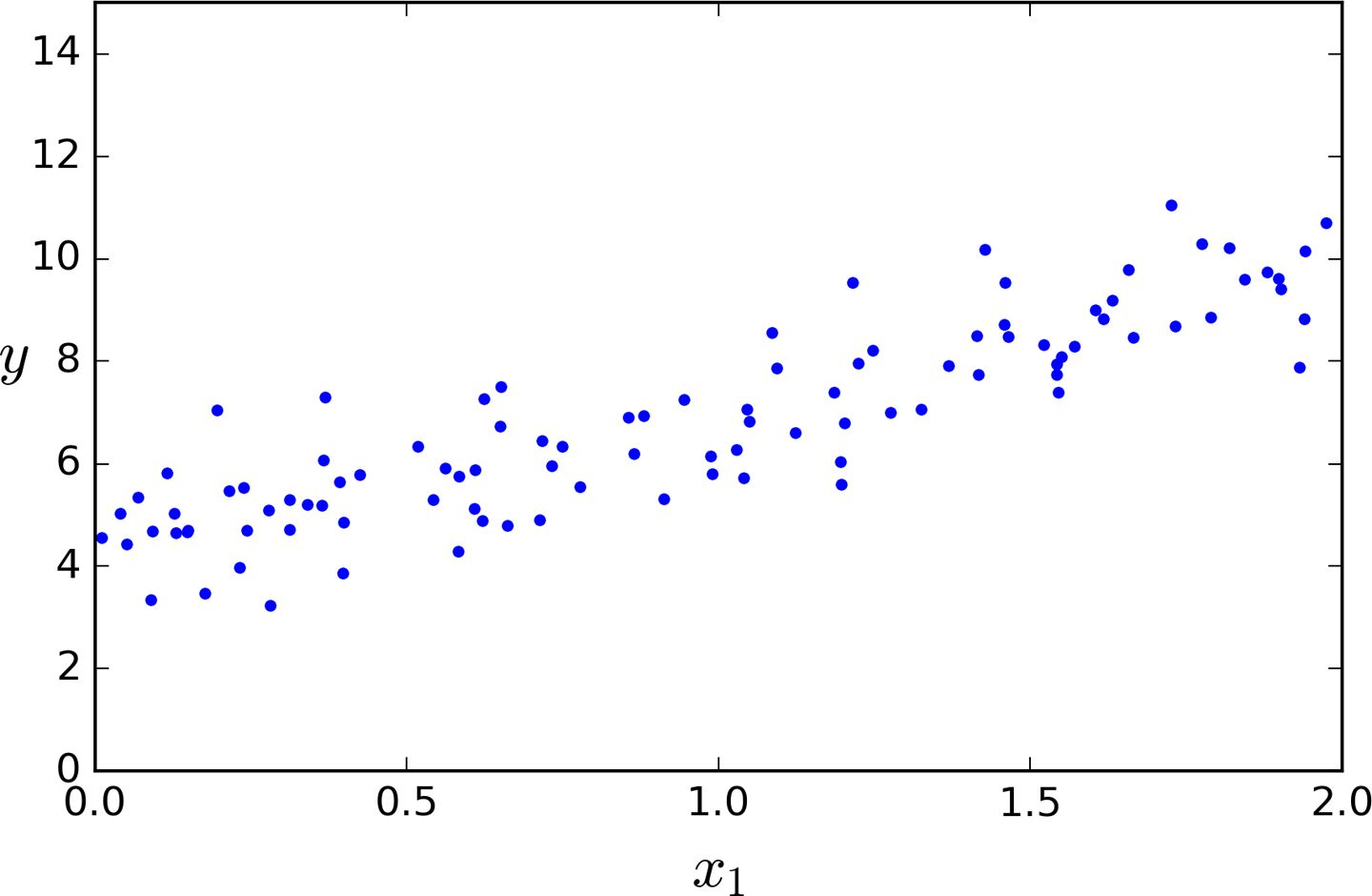X_b = np.c_[np.ones((100, 1)), X]
theta_best = np.linalg.inv(X_b.T.dot(X_B)).dot(X_b.T).dot(y)


>>> theta_best
array([[4.21509616],[2.77011339]])


>>> X_new = np.array([,])
>>> X_new_b = np.c_[np.ones((2, 1)), X_new]
>>> y_predict = X_new_b.dot(theta.best)
>>> y_predict
array([[4.21509616],[9.75532293]])


plt.plot(X_new,y_predict,"r-")
plt.plot(X,y,"b.")
plt.axis([0,2,0,15])
plt.show()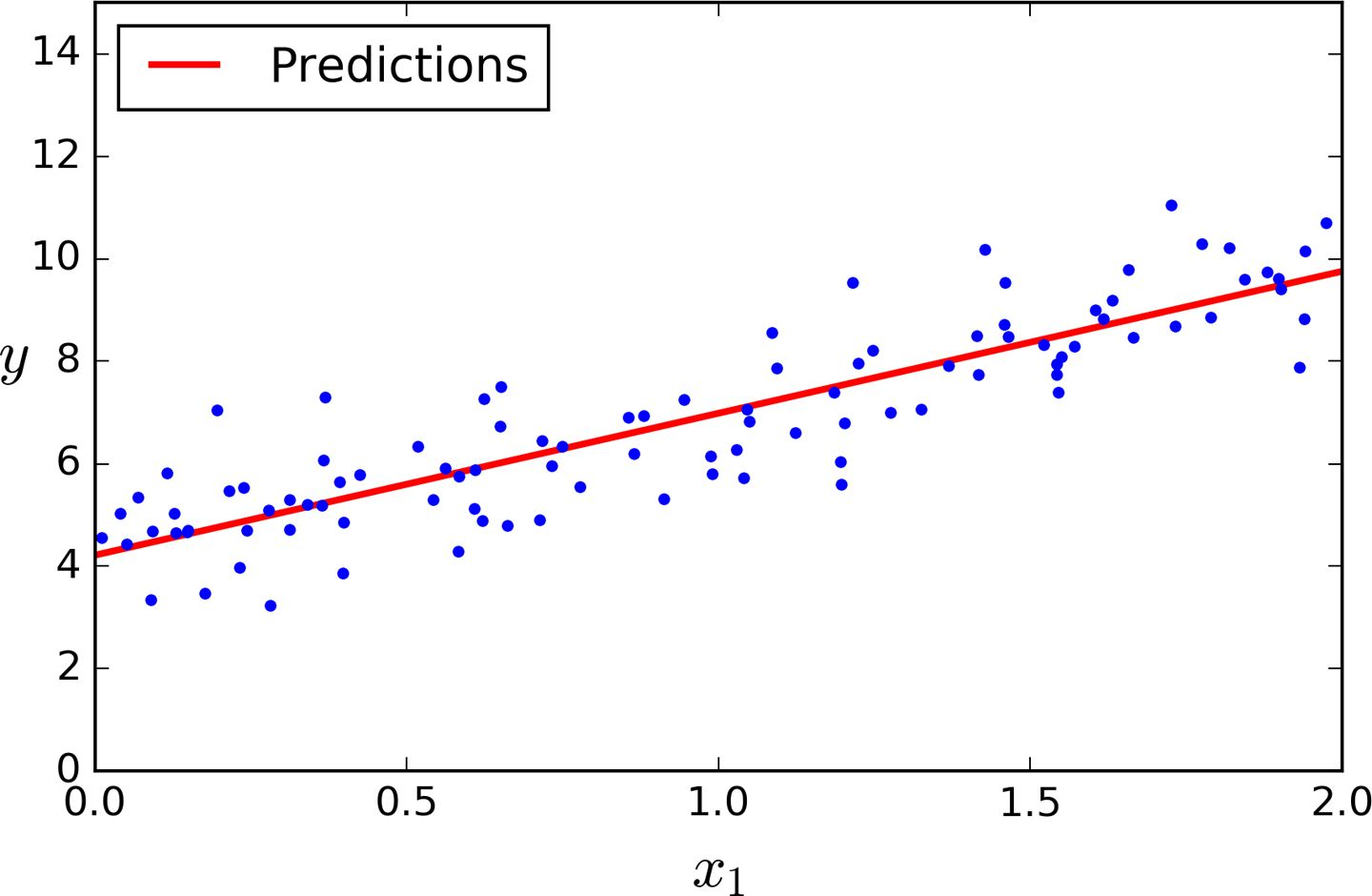>>> form sklearn.linear_model import LinearRegression
>>> lin_reg = LinearRegression()
>>> lin_reg.fit(X,y)
>>> lin_reg.intercept_, lin_reg.coef_
(array([4.21509616]),array([2.77011339]))
>>> lin_reg.predict(X_new)
array([[4.21509616],[9.75532293]])


## 梯度下降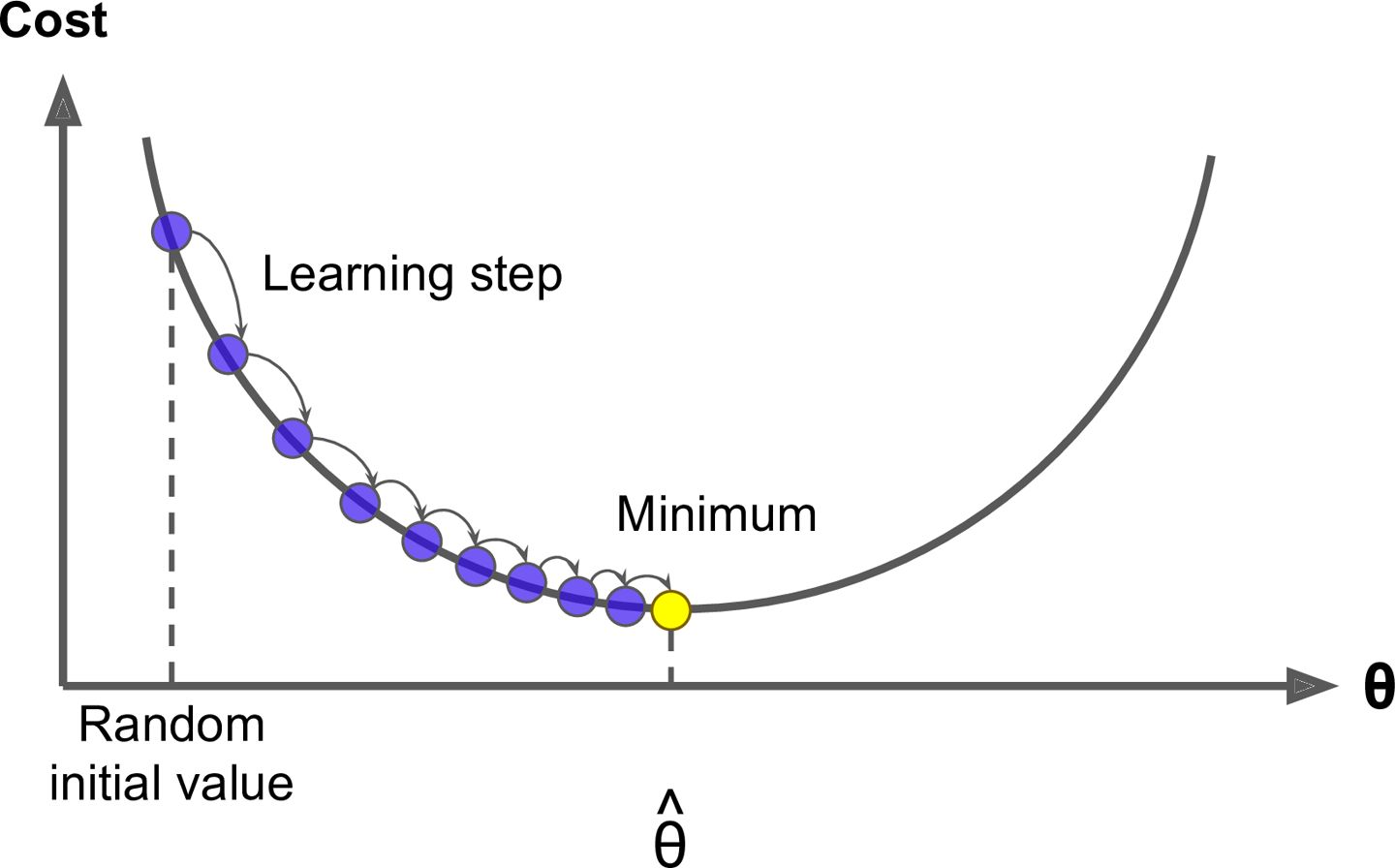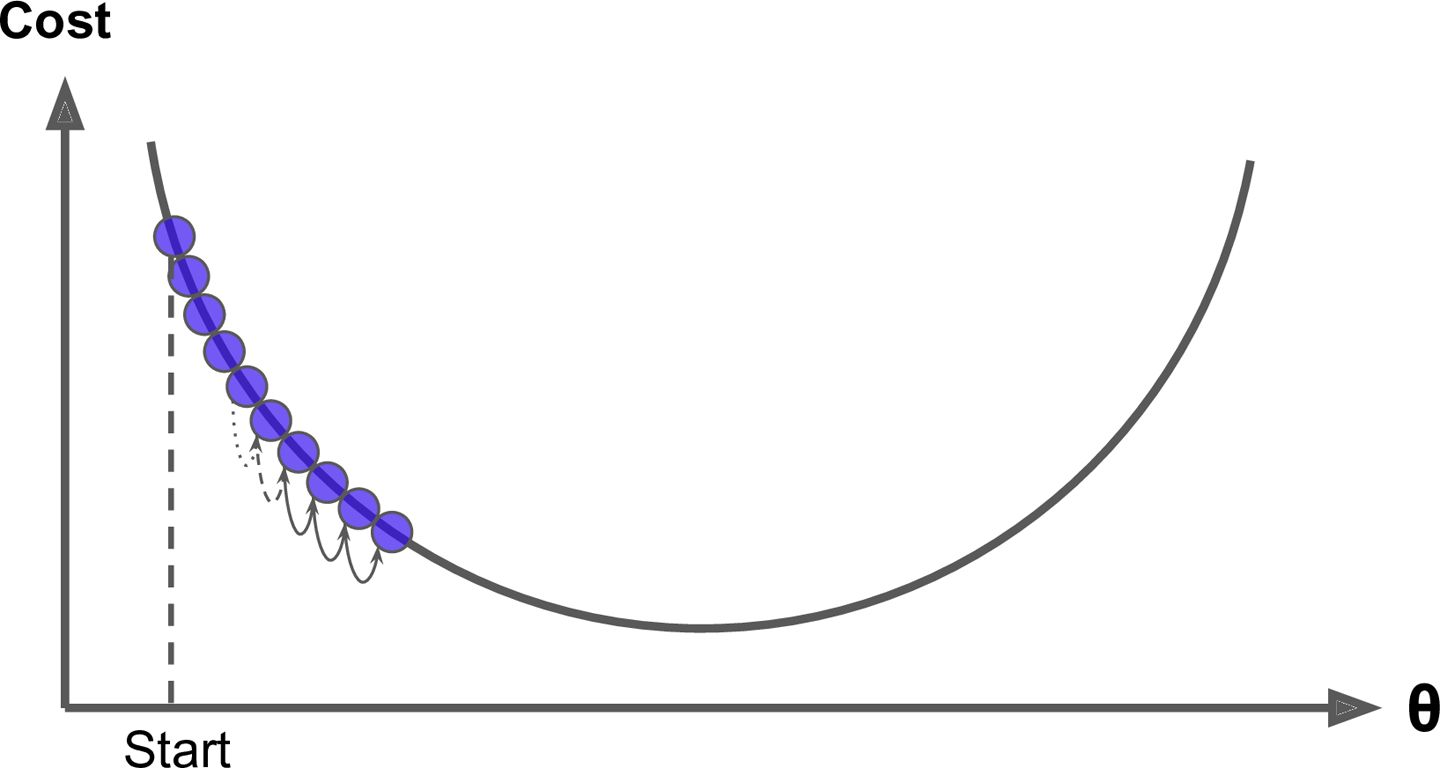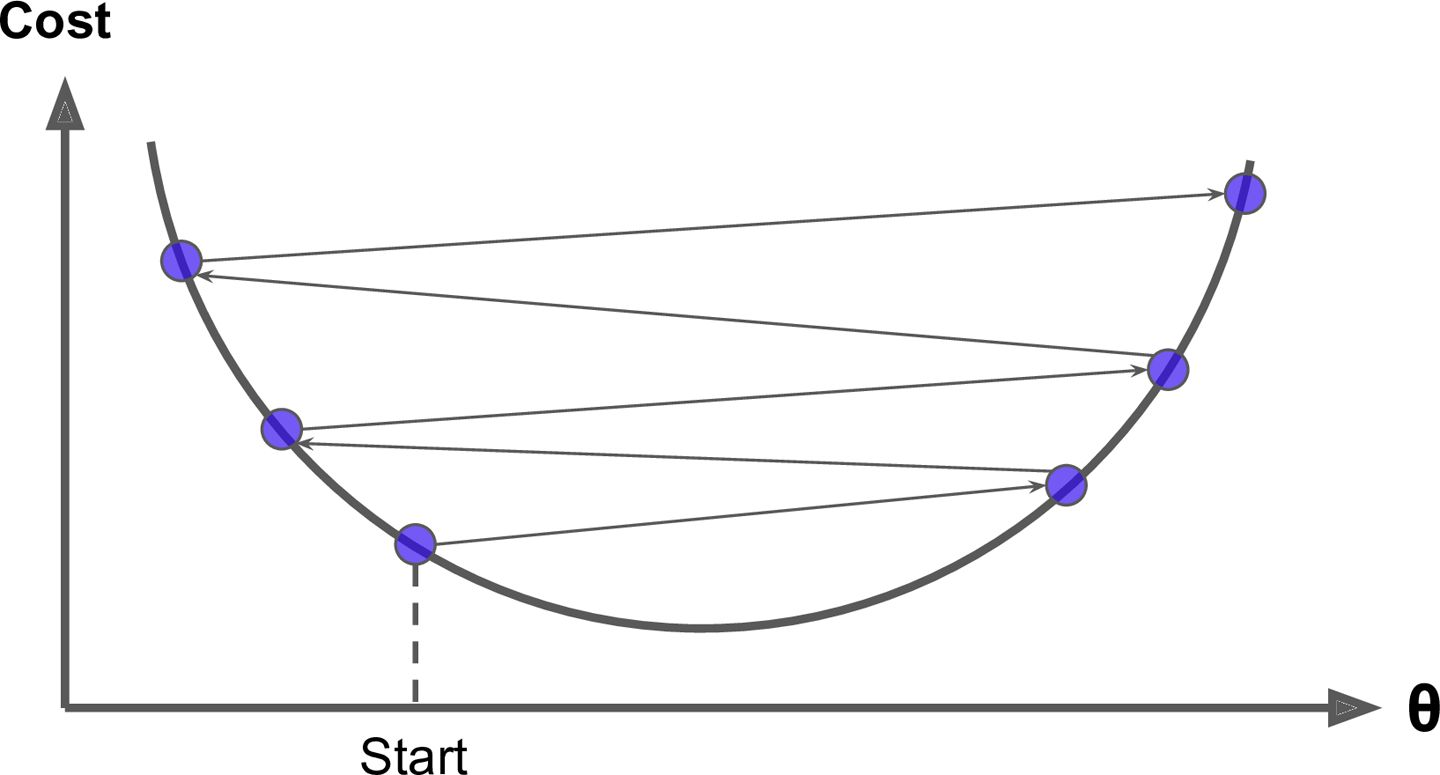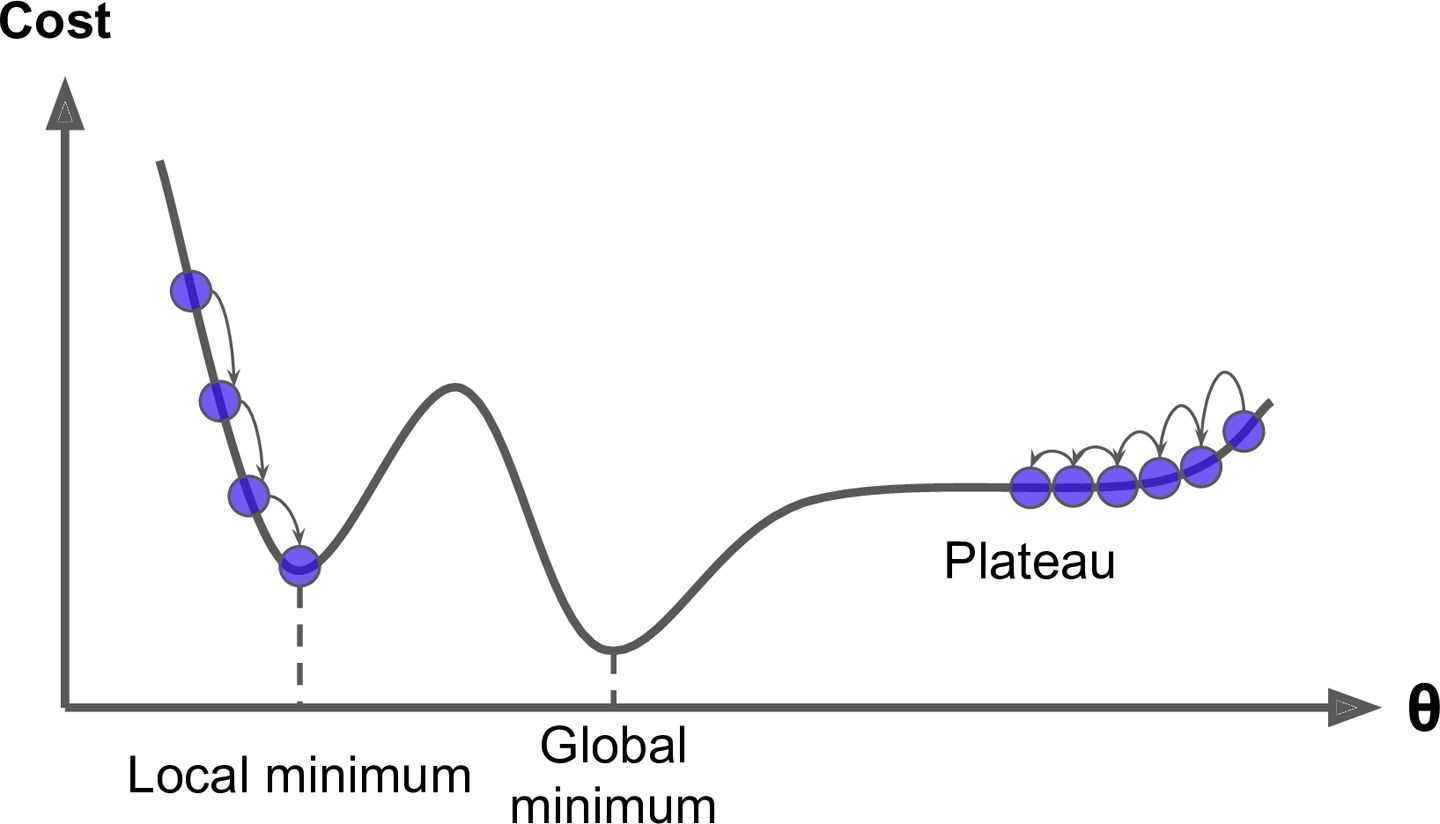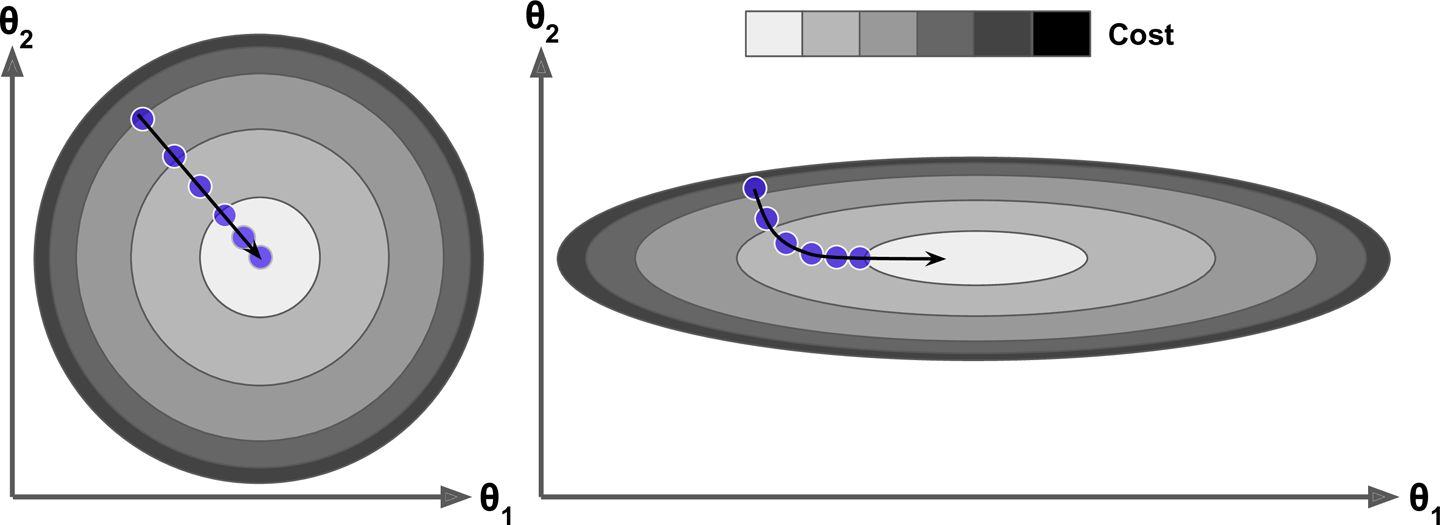### 批量梯度下降eta = 0.1 # 学习率
n_iterations = 1000
m = 100

theta = np.random.randn(2,1) # 随机初始值

for iteration in range(n_iterations):
gradients = 2/m * X_b.T.dot(X_b.dot(theta) - y)
theta = theta - eta * gradiens


>>> theta
array([[4.21509616],[2.77011339]])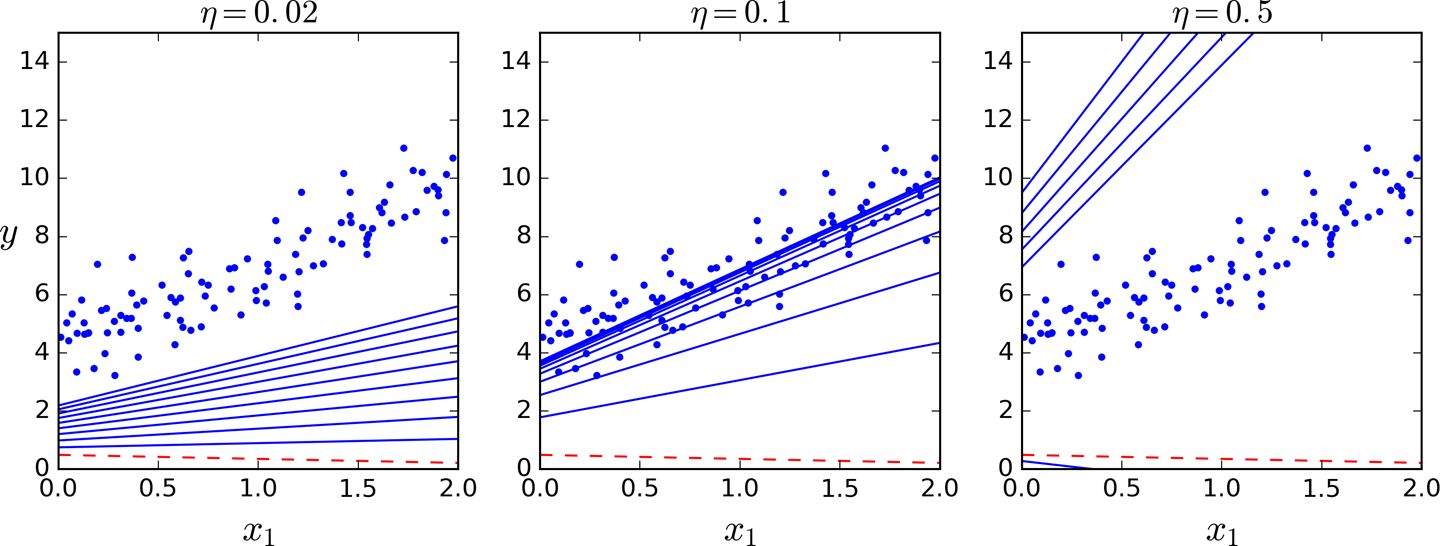### 随机梯度下降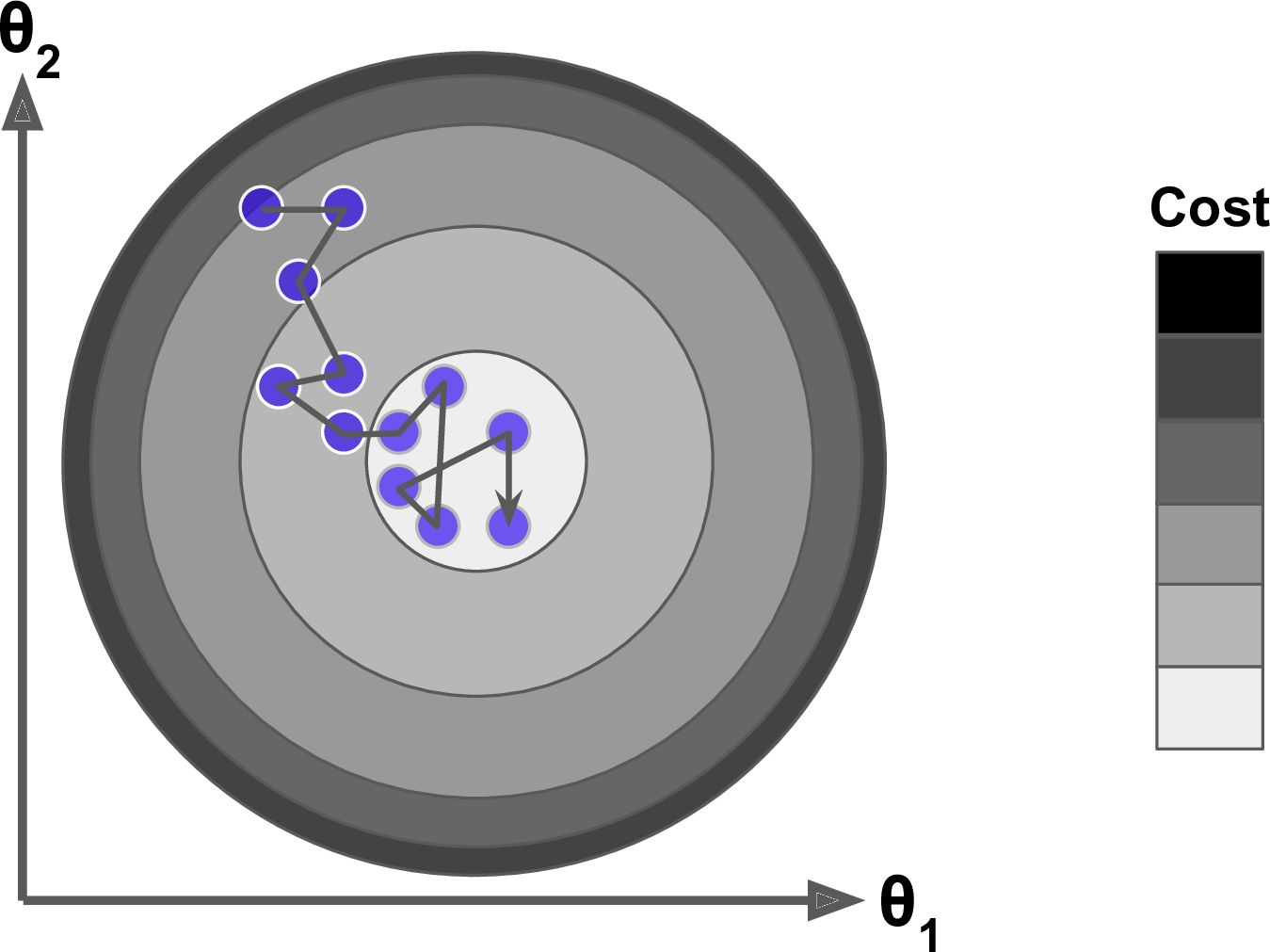n_epochs = 50
t0, t1 = 5, 50  #learning_schedule的超参数

def learning_schedule(t):
return t0 / (t + t1)

theta = np.random.randn(2,1)

for epoch in range(n_epochs):
for i in range(m):
random_index = np.random.randint(m)
xi = X_b[random_index:random_index+1]
yi = y[random_index:random_index+1]
eta = learning_schedule(epoch * m + i)
theta = theta - eta * gradiens


>>> theta
array([[4.21076011],[2.748560791]])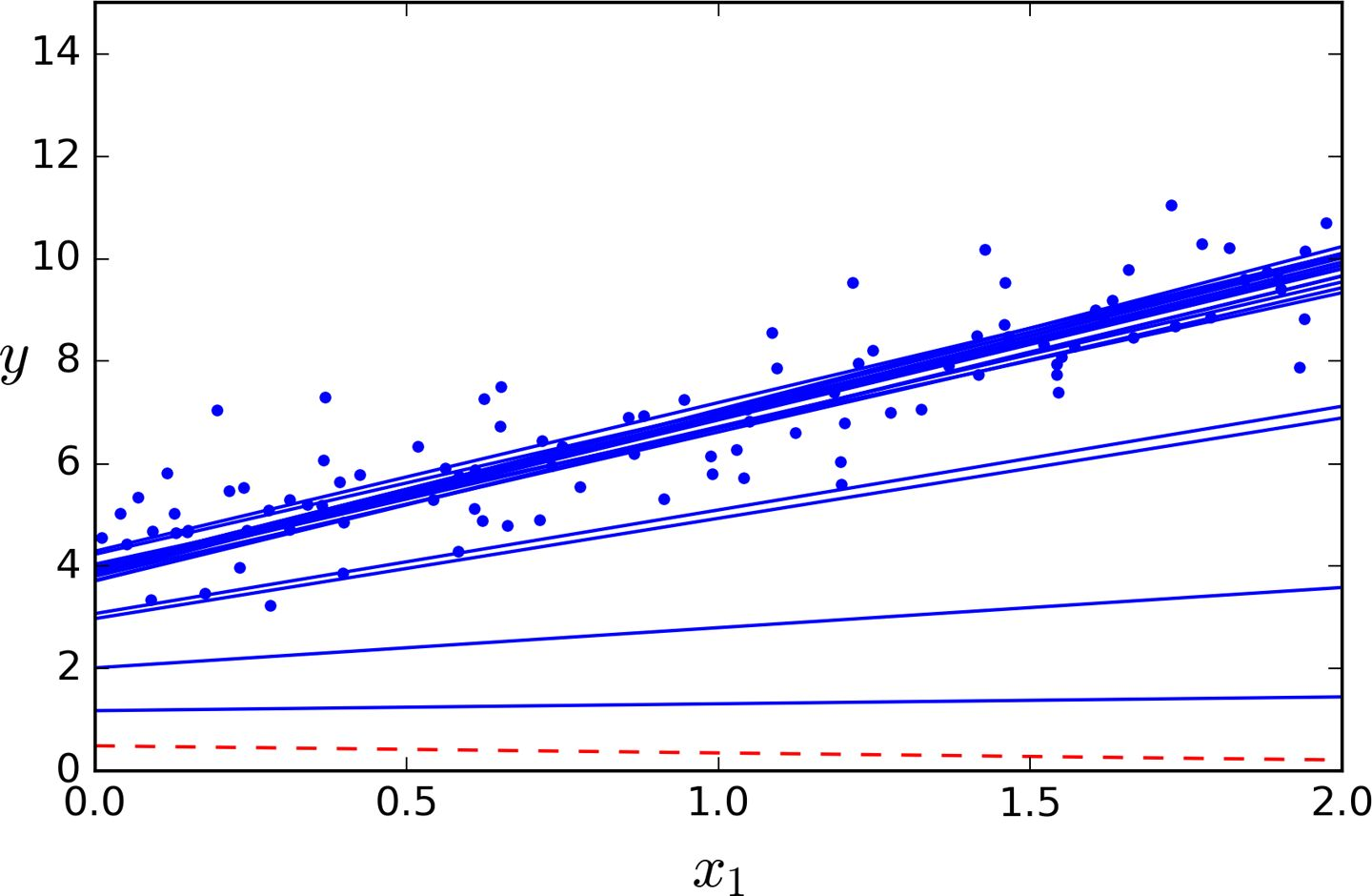from sklearn.linear_model import SGDRegressor
sgd_reg + SGDRregressor(n_iter=50, penalty=None, eta0=0.1)
sgd_reg.fit(X,y.ravel())


>>> sgd_reg.intercept_, sgd_reg.coef_
(array([4.18380366]),array([2.74205299]))


### 小批量梯度下降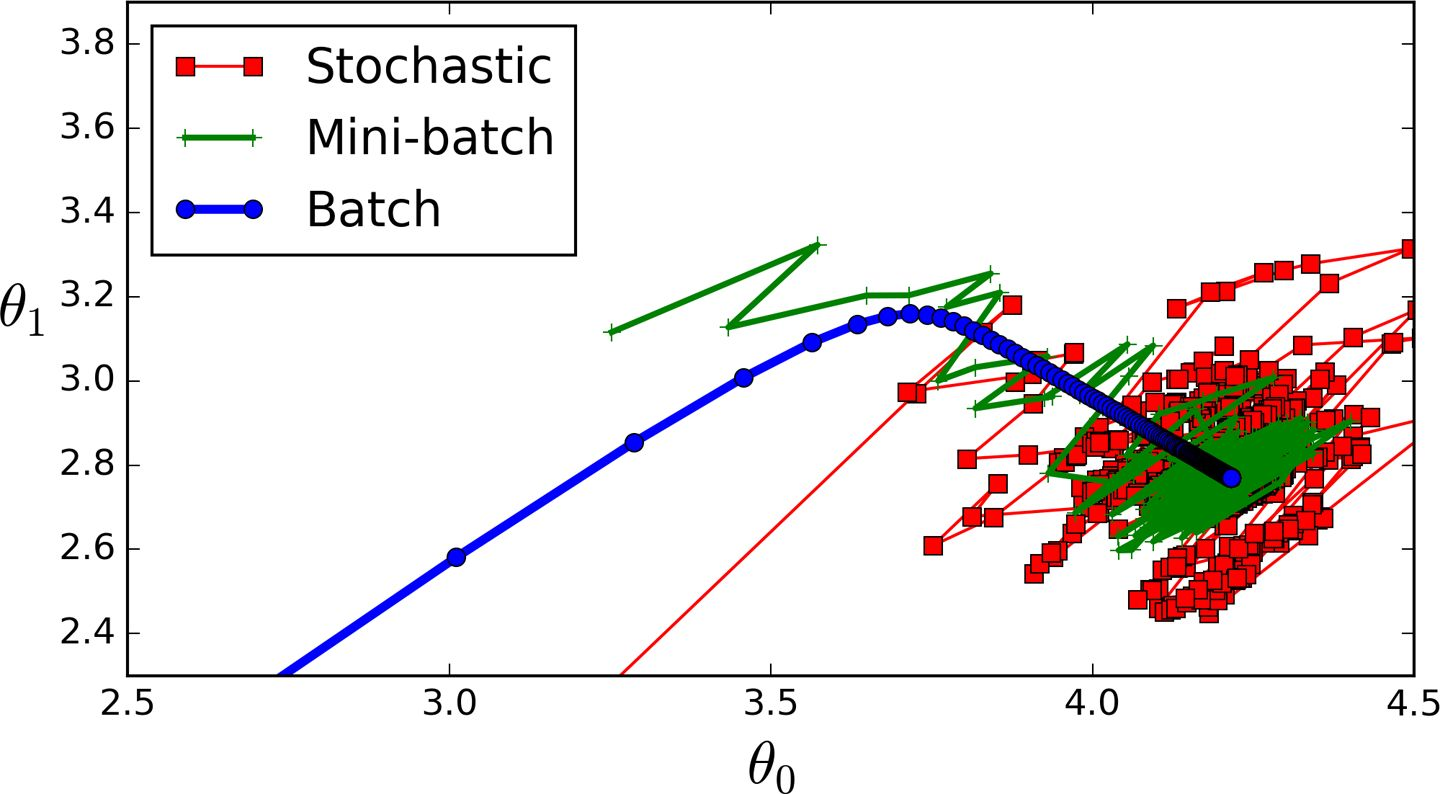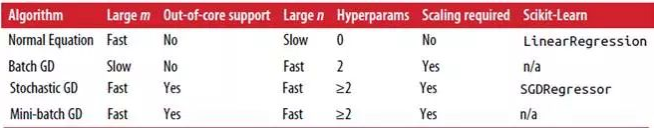## 多项式回归

m = 100
X = 6 * np.random.rand(m, 1) - 3
y = 0.5 * X**2 + X + 2 + np.random.randn(m, 1)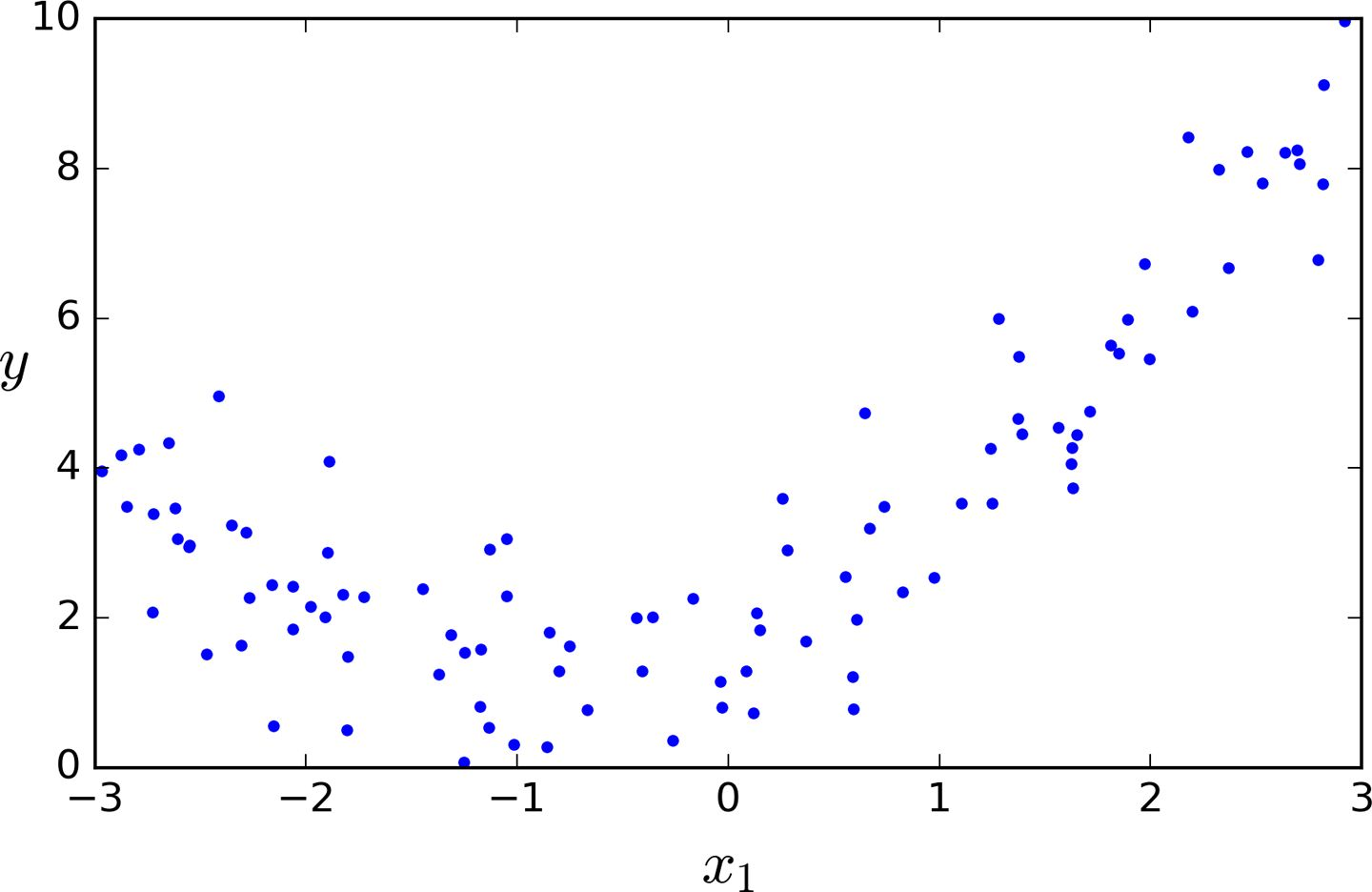>>> from sklearn.preprocessing import PolynomialFeatures
>>> poly_features = PolynomialFeatures(degree=2,include_bias=False)
>>> X_poly = poly_features.fit_transform(X)
>>> X
array([-0.75275929])
>>> X_poly
array([-0.75275929, 0.56664654])


X_poly现在包含原始特征X并加上了这个特征的平方X2。现在你可以在这个扩展训练集上使用LinearRegression模型进行拟合，如图 4-13：

>>> lin_reg = LinearRegression()
>>> lin_reg.fit(X_poly, y)
>>> lin_reg.intercept_, lin_reg.coef_
(array([ 1.78134581]), array([[ 0.93366893, 0.56456263]]))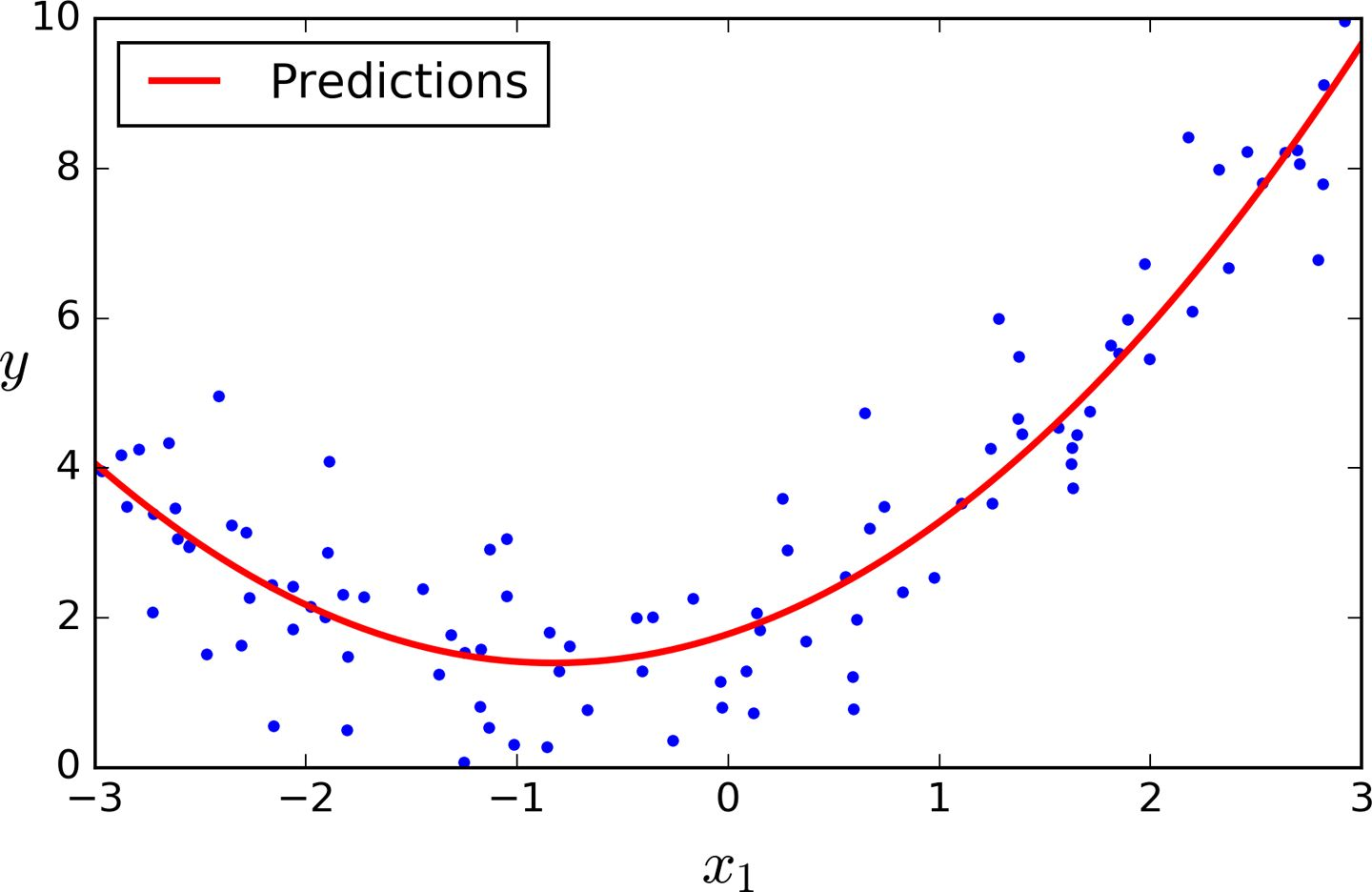PolynomialFeatures(degree=d)把一个包含n个特征的数组转换为一个包含(n+d)!/d!n!个特征的数组，n!是n的阶乘，等于1 × 2 × 3 × ⋯ × n。小心大量特征的组合爆炸！

## 学习曲线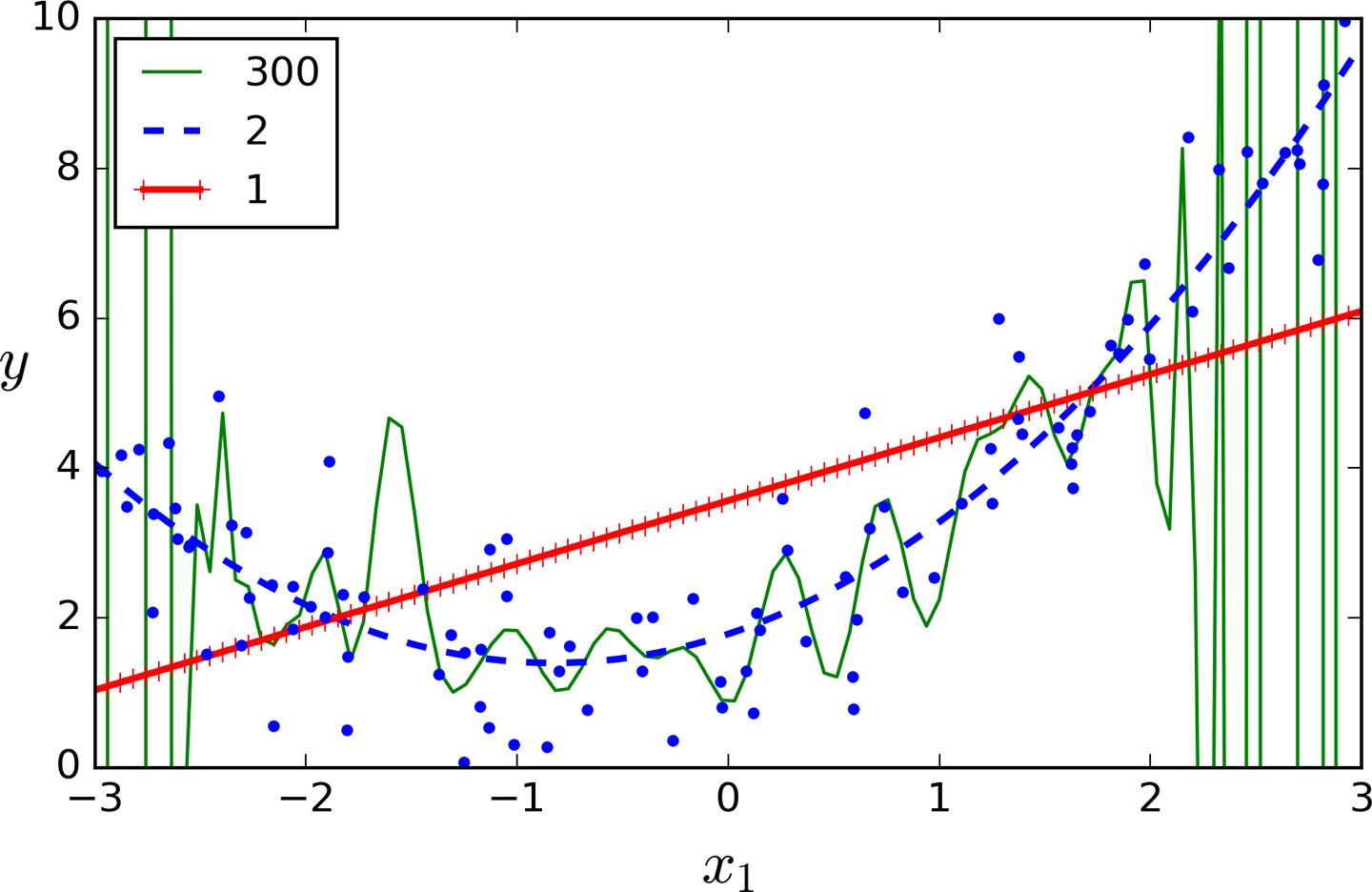from sklearn.metrics import mean_squared_error
from sklearn.model_selection import train_test_split

def plot_learning_curves(model, X, y):
X_train, X_val, y_train, y_val = train_test_split(X, y, test_size=0.2)
train_errors, val_errors = [], []
for m in range(1, len(X_train)):
model.fit(X_train[:m], y_train[:m])
y_train_predict = model.predict(X_train[:m])
y_val_predict = model.predict(X_val)
train_errors.append(mean_squared_error(y_train_predict, y_train[:m]))
val_errors.append(mean_squared_error(y_val_predict, y_val))
plt.plot(np.sqrt(train_errors), "r-+", linewidth=2, label="train")
plt.plot(np.sqrt(val_errors), "b-", linewidth=3, label="val")


lin_reg = LinearRegression()
plot_learning_curves(lin_reg, X, y)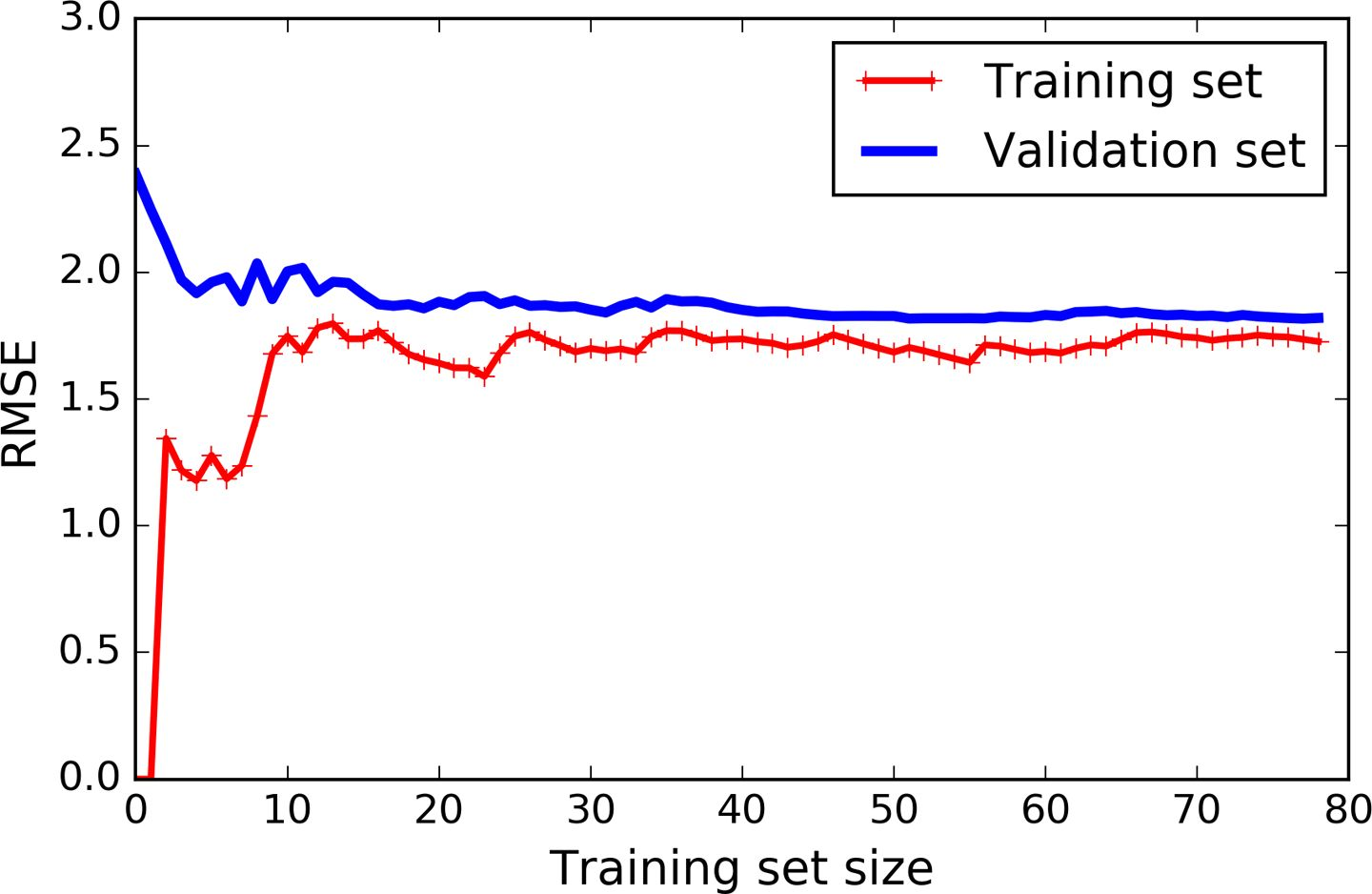from sklearn.pipeline import Pipeline

polynomial_regression = Pipeline((
("poly_features", PolynomialFeatures(degree=10, include_bias=False)),
("sgd_reg", LinearRegression()),
))

plot_learning_curves(polynomial_regression, X, y)


• 在训练集上，误差要比线性回归模型低的多。
• 图中的两条曲线之间有间隔，这意味模型在训练集上的表现要比验证集上好的多，这也是模型过拟合的显著特点。当然，如果你使用了更大的训练数据，这两条曲线最后会非常的接近。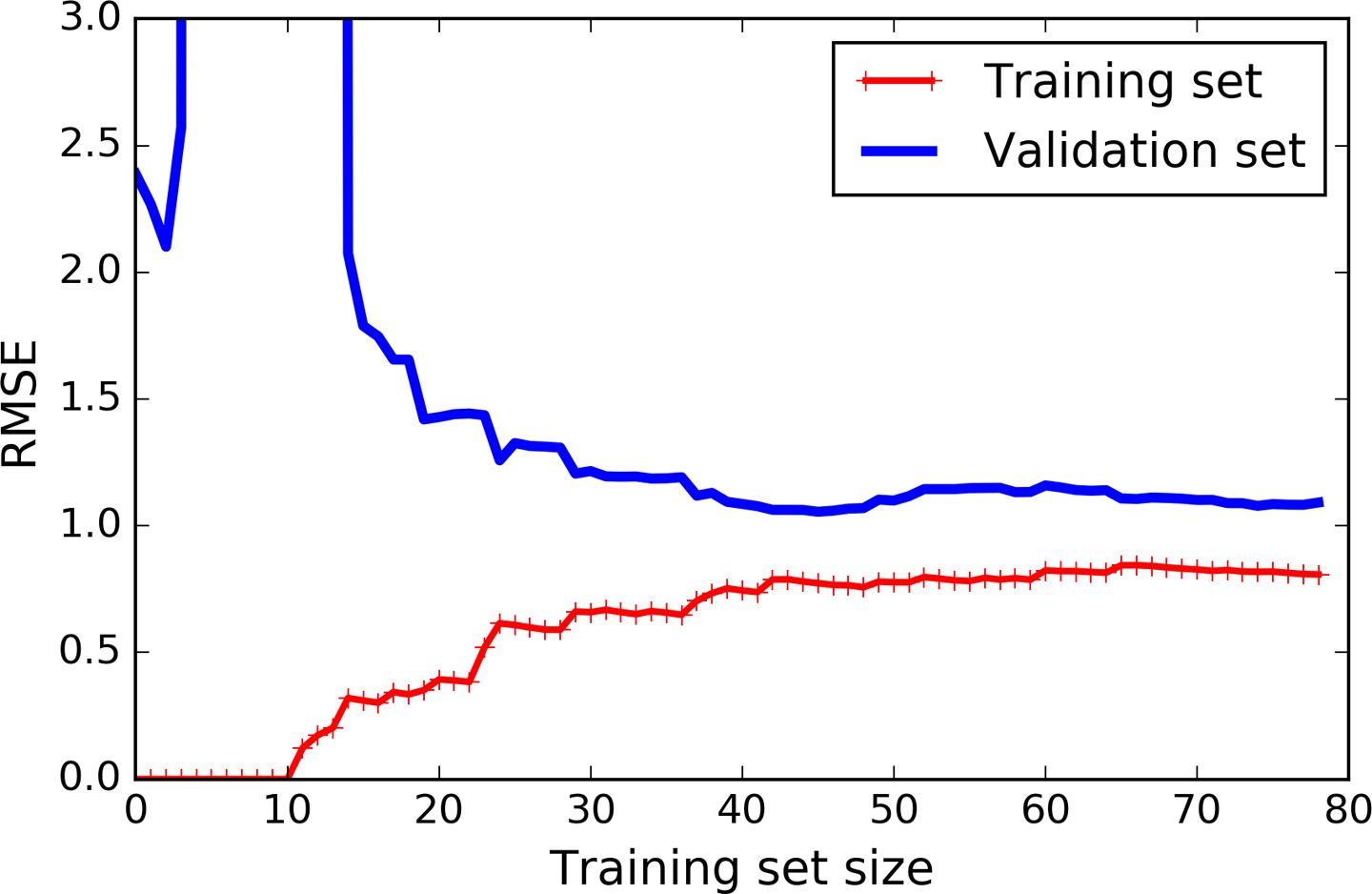• 偏差：泛化误差的这部分误差是由于错误的假设决定的。例如实际是一个二次模型，你却假设了一个线性模型。一个高偏差的模型最容易出现欠拟合。
• 方差：这部分误差是由于模型对训练数据的微小变化较为敏感，一个多自由度的模型更容易有高的方差（例如一个高阶多项式模型），因此会导致模型过拟合。
• 不可约误差：这部分误差是由于数据本身的噪声决定的。降低这部分误差的唯一方法就是进行数据清洗（例如：修复数据源，修复坏的传感器，识别和剔除异常值）。

## 线性模型的正则化

### 岭（Ridge）回归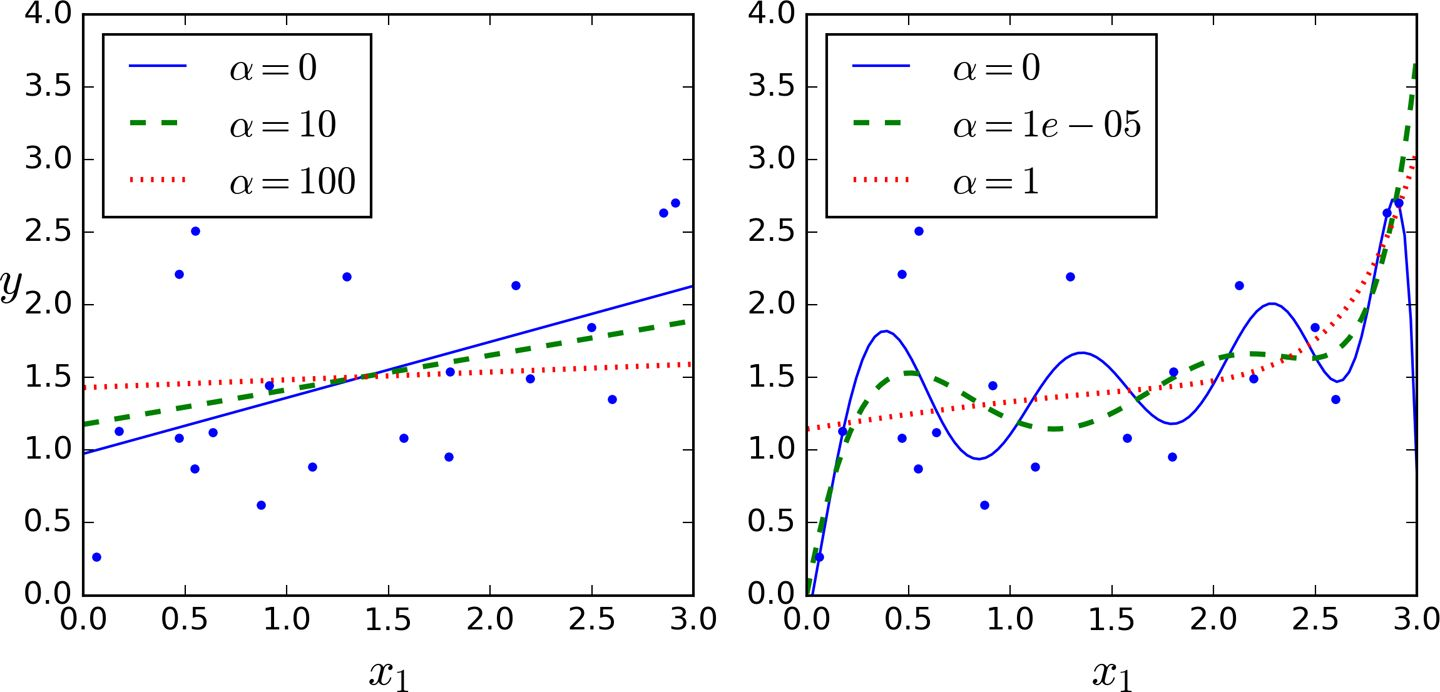>>> from sklearn.linear_model import Ridge
>>> ridge_reg = Ridge(alpha=1, solver="cholesky")
>>> ridge_reg.fit(X, y)
>>> ridge_reg.predict([[1.5]])
array([[ 1.55071465]]


>>> sgd_reg = SGDRegressor(penalty="l2")
>>> sgd_reg.fit(X, y.ravel())
>>> sgd_reg.predict([[1.5]])
array([[ 1.13500145]])


penalty参数指的是正则项的惩罚类型。指定“l2”表明你要在损失函数上添加一项：权重向量范数平方的一半，这就是简单的岭回归。

### Lasso 回归

Lasso 回归（也称 Least Absolute Shrinkage，或者 Selection Operator Regression）是另一种正则化版的线性回归：就像岭回归那样，它也在损失函数上添加了一个正则化项，但是它使用权重向量的范数而不是权重向量范数平方的一半。（如公式 4-10）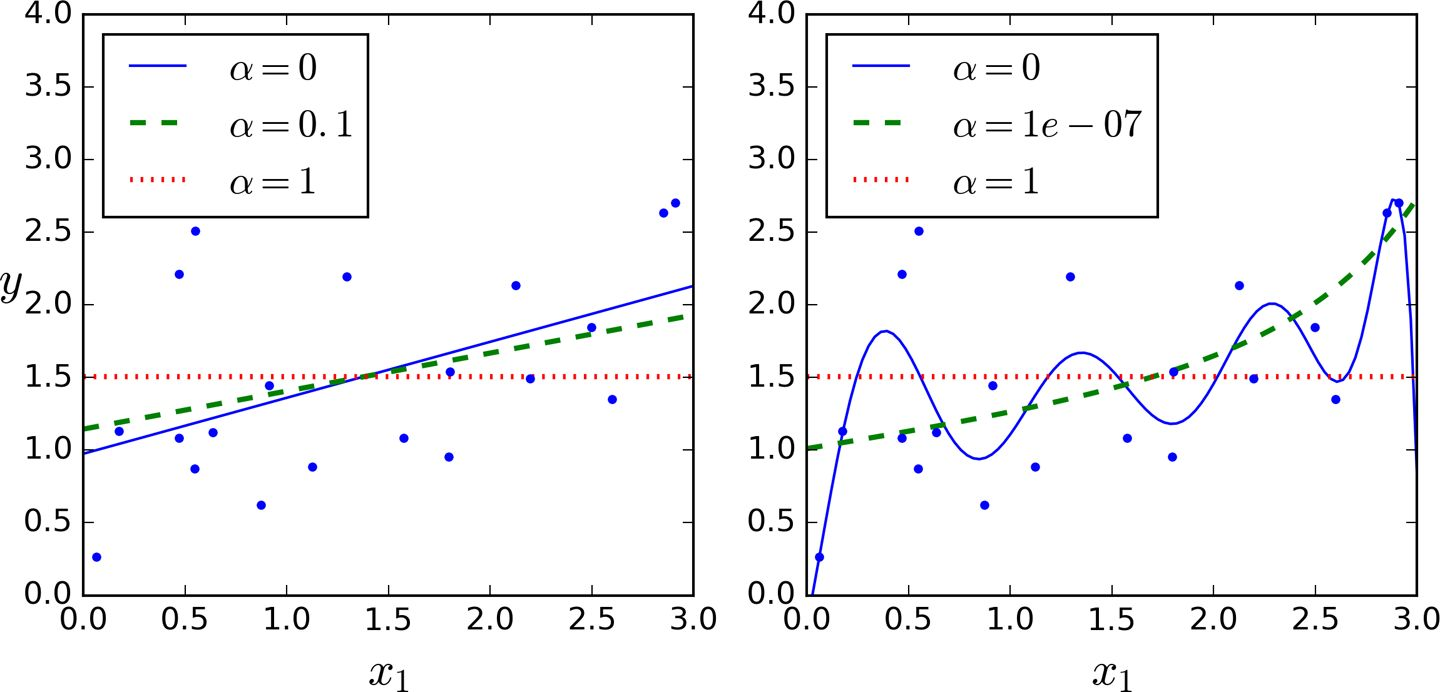Lasso 回归的一个重要特征是它倾向于完全消除最不重要的特征的权重（即将它们设置为零）。例如，右图中的虚线所示（），曲线看起来像一条二次曲线，而且几乎是线性的，这是因为所有的高阶多项特征都被设置为零。换句话说，Lasso回归自动的进行特征选择同时输出一个稀疏模型（即，具有很少的非零权重）。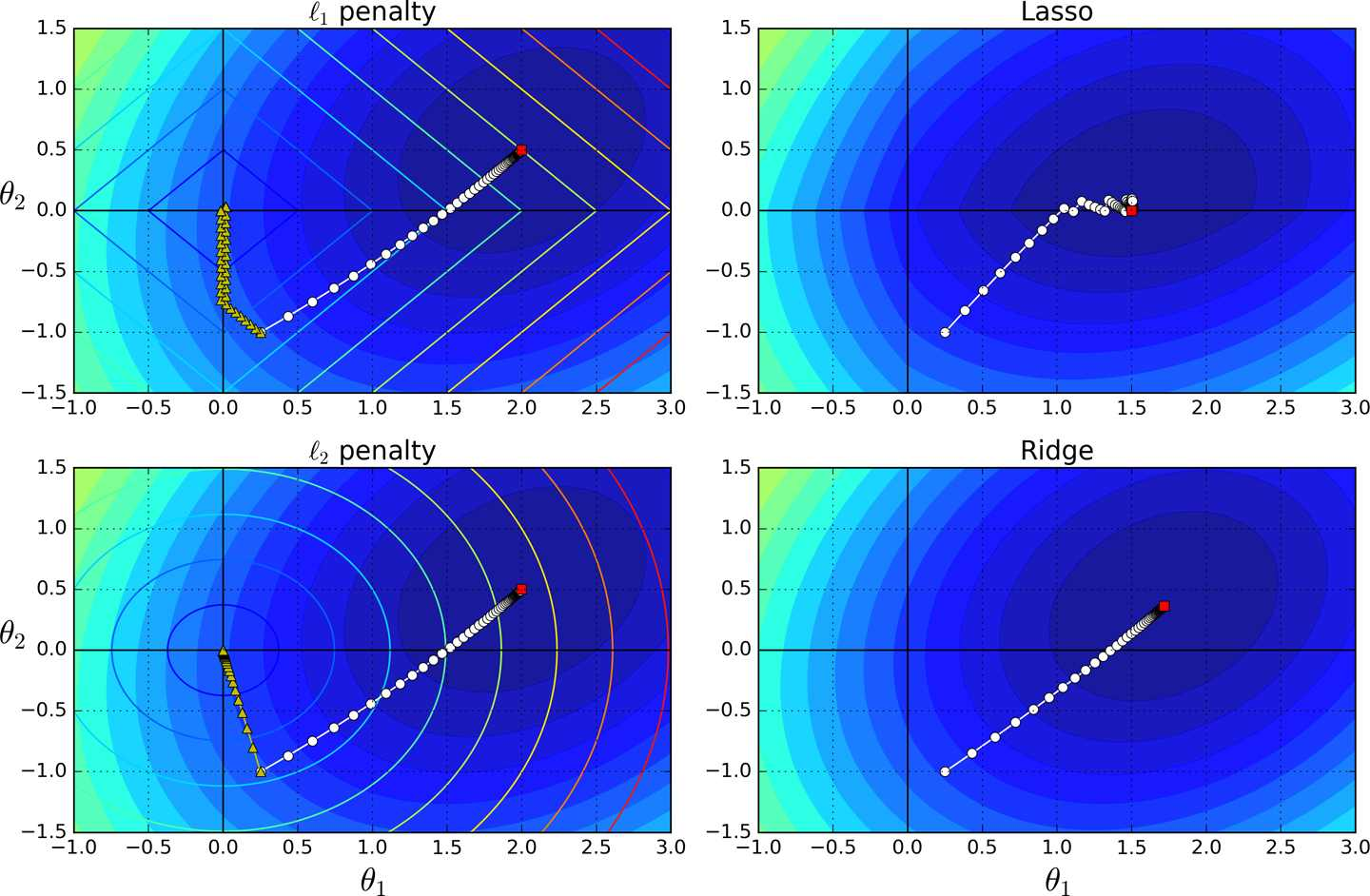Lasso 损失函数在处无法进行微分运算，但是梯度下降如果你使用子梯度向量后它可以在任何的情况下进行计算。公式 4-11 是在 Lasso 损失函数上进行梯度下降的子梯度向量公式。>>> from sklearn.linear_model import Lasso
>>> lasso_reg = Lasso(alpha=0.1)
>>> lasso_reg.fit(X, y)
>>> lasso_reg.predict([[1.5]])
array([ 1.53788174]


### 弹性网络（ElasticNet）>>> from sklearn.linear_model import ElasticNet
>>> elastic_net = ElasticNet(alpha=0.1, l1_ratio=0.5)
>>> elastic_net.fit(X, y)
>>> elastic_net.predict([[1.5]])
array([ 1.54333232])


### 早期停止法（Early Stopping）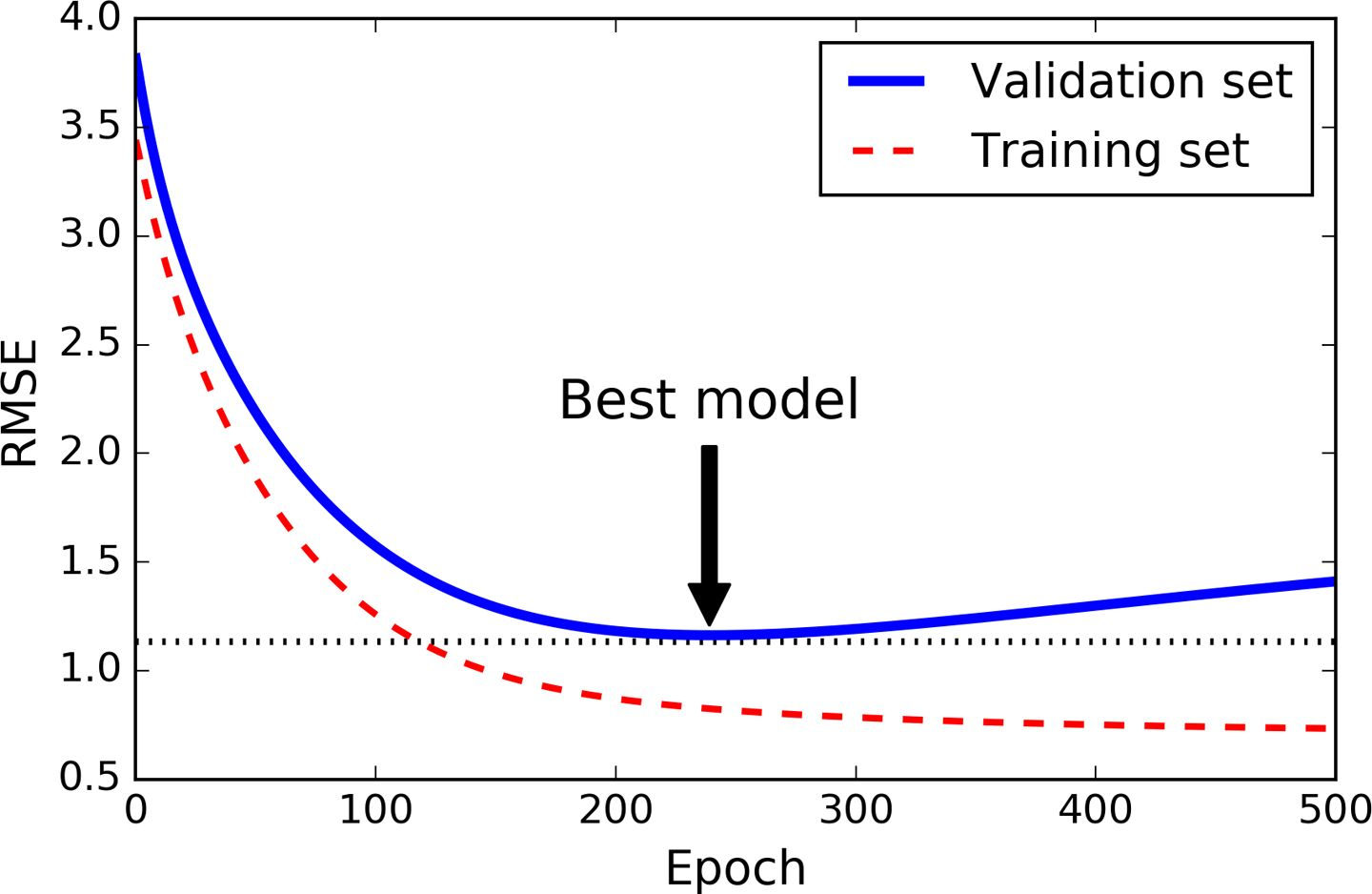from sklearn.base import clone
sgd_reg = SGDRegressor(n_iter=1, warm_start=True, penalty=None,learning_rate="constant", eta0=0.0005)

minimum_val_error = float("inf")
best_epoch = None
best_model = None
for epoch in range(1000):
sgd_reg.fit(X_train_poly_scaled, y_train)
y_val_predict = sgd_reg.predict(X_val_poly_scaled)
val_error = mean_squared_error(y_val_predict, y_val)
if val_error < minimum_val_error:
minimum_val_error = val_error
best_epoch = epoch
best_model = clone(sgd_reg)


## 逻辑回归

### 概率估计Logistic 函数（也称为 logit），用表示，其是一个 sigmoid 函数（图像呈 S 型），它的输出是一个介于 0 和 1 之间的数字。其定义如公式 4-14 和图 4-21 所示。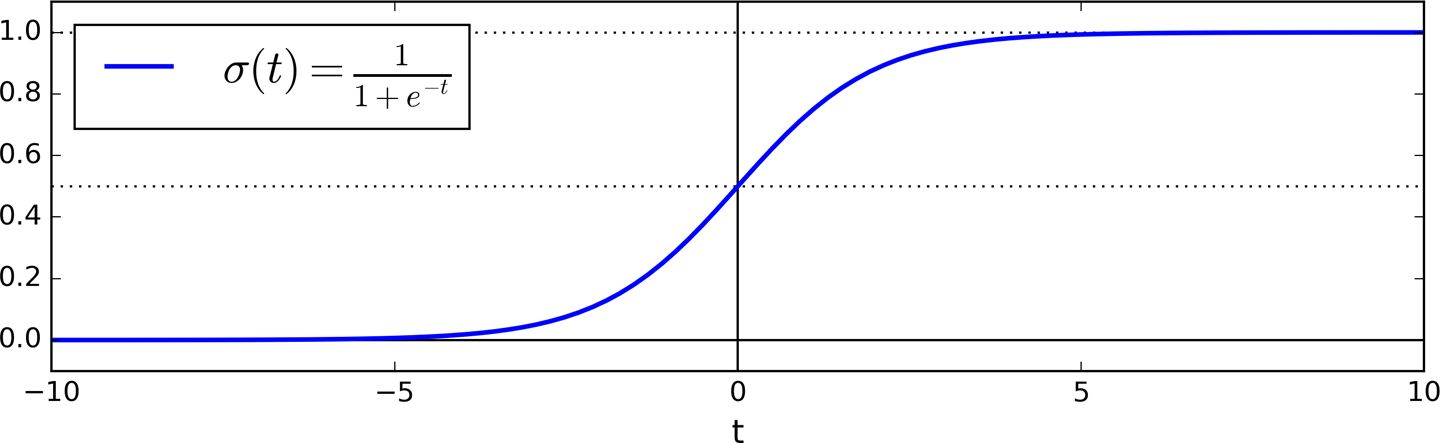### 训练和损失函数### 决策边界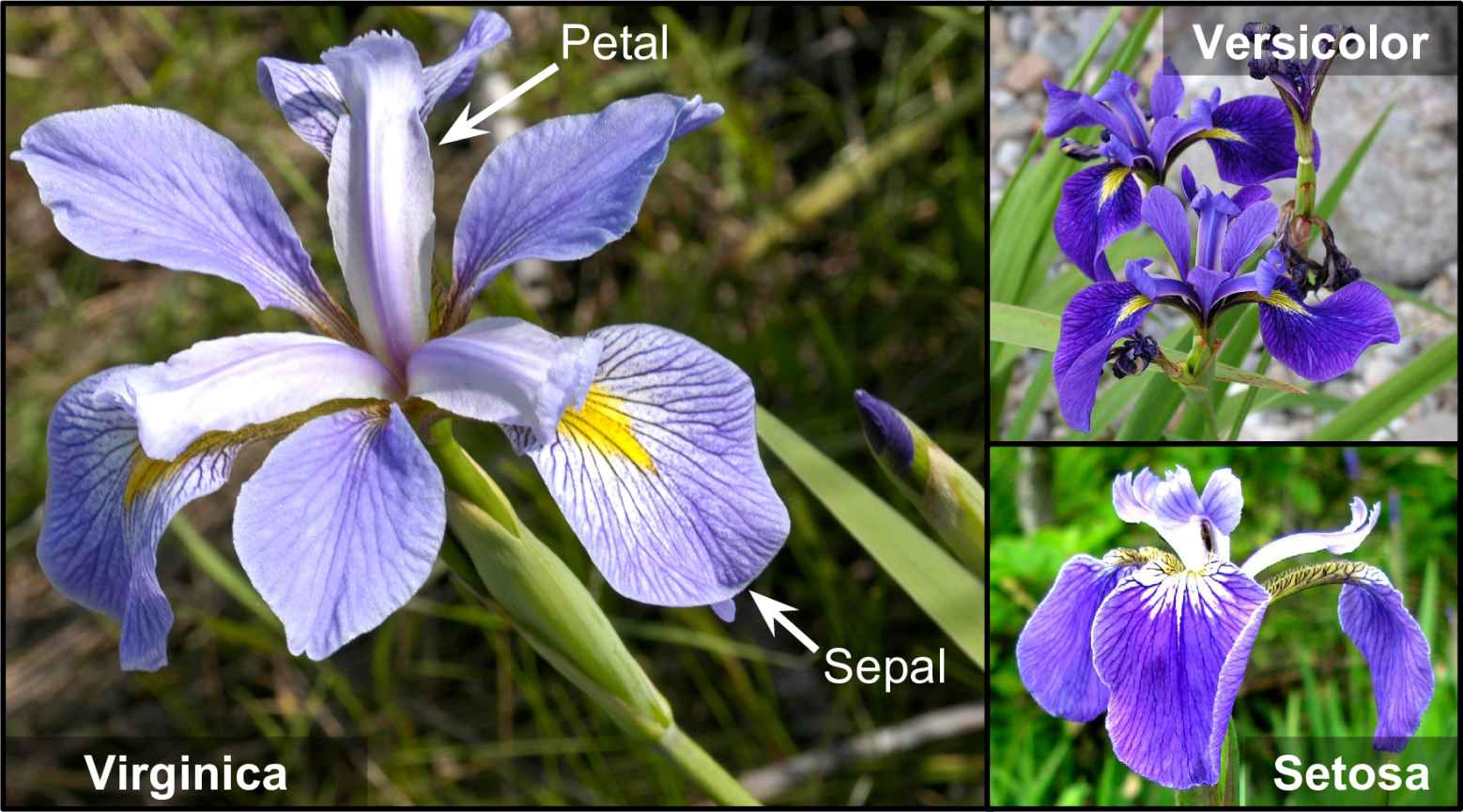>>> from sklearn import datasets
>>> list(iris.keys())
['data', 'target_names', 'feature_names', 'target', 'DESCR']
>>> X = iris["data"][:, 3:] # 花瓣宽度
>>> y = (iris["target"] == 2).astype(np.int)


from sklearn.linear_model import LogisticRegression

log_reg = LogisticRegression()
log_reg.fit(X, y)


X_new = np.linspace(0, 3, 1000).reshape(-1, 1)
y_proba = log_reg.predict_proba(X_new)
plt.plot(X_new, y_proba[:, 1], "g-", label="Iris-Virginica")
plt.plot(X_new, y_proba[:, 0], "b--", label="Not Iris-Virginica"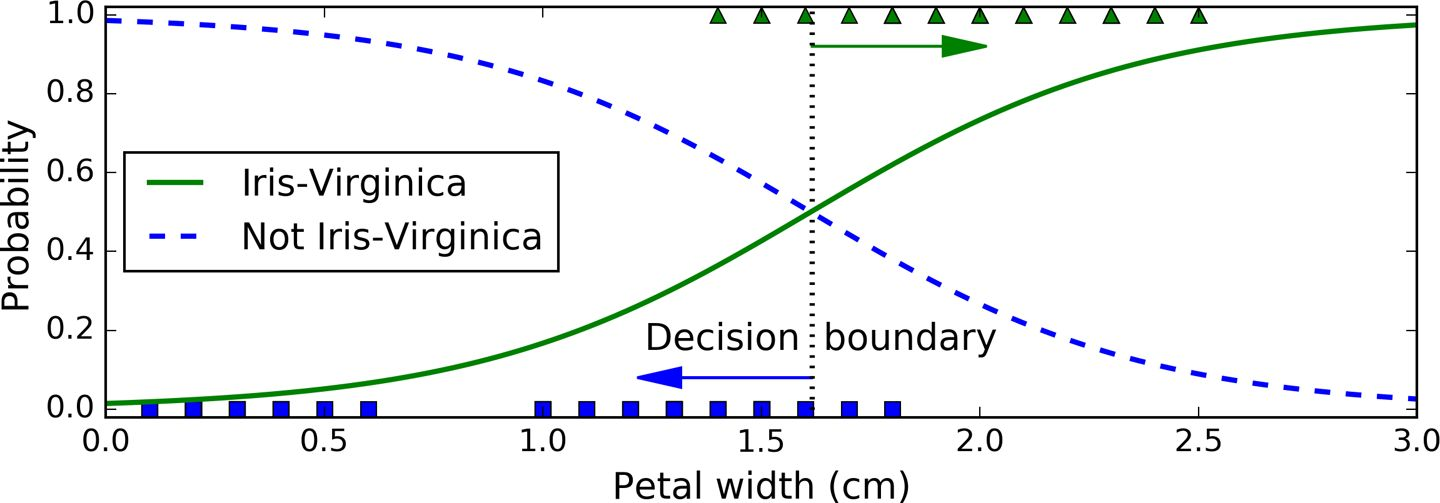Virginica 花的花瓣宽度（用三角形表示）在 1.4 厘米到 2.5 厘米之间，而其他种类的花（由正方形表示）通常具有较小的花瓣宽度，范围从 0.1 厘米到 1.8 厘米。注意，它们之间会有一些重叠。在大约 2 厘米以上时，分类器非常肯定这朵花是Virginica花（分类器此时输出一个非常高的概率值），而在1厘米以下时，它非常肯定这朵花不是 Virginica 花（不是 Virginica 花有非常高的概率）。在这两个极端之间，分类器是不确定的。但是，如果你使用它进行预测（使用predict()方法而不是predict_proba()方法），它将返回一个最可能的结果。因此，在 1.6 厘米左右存在一个决策边界，这时两类情况出现的概率都等于 50%：如果花瓣宽度大于 1.6 厘米，则分类器将预测该花是 Virginica，否则预测它不是（即使它有可能错了）：

>>> log_reg.predict([[1.7], [1.5]])
array([1, 0])### Softmax 回归

Logistic 回归模型可以直接推广到支持多类别分类，不必组合和训练多个二分类器（如第 3 章所述）， 其称为 Softmax 回归或多类别 Logistic 回归。•是类的个数
•是实例的每个类的得分的向量
•表示给定每一类分数之后，实例属于第类的概率• argmax运算返回一个函数取到最大值的变量值。 在这个等式，它返回使最大时的的值

Softmax 回归分类器一次只能预测一个类（即它是多类的，但不是多输出的），因此它只能用于判断互斥的类别，如不同类型的植物。 你不能用它来识别一张照片中的多个人。• 如果对于第个实例的目标类是，那么，反之X = iris["data"][:, (2, 3)] # petal length, petal width
y = iris["target"]

softmax_reg = LogisticRegression(multi_class="multinomial",solver="lbfgs", C=10)
softmax_reg.fit(X, y)


>>> softmax_reg.predict([[5, 2]])
array()
>>> softmax_reg.predict_proba([[5, 2]])
array([[ 6.33134078e-07, 5.75276067e-02, 9.42471760e-01]])是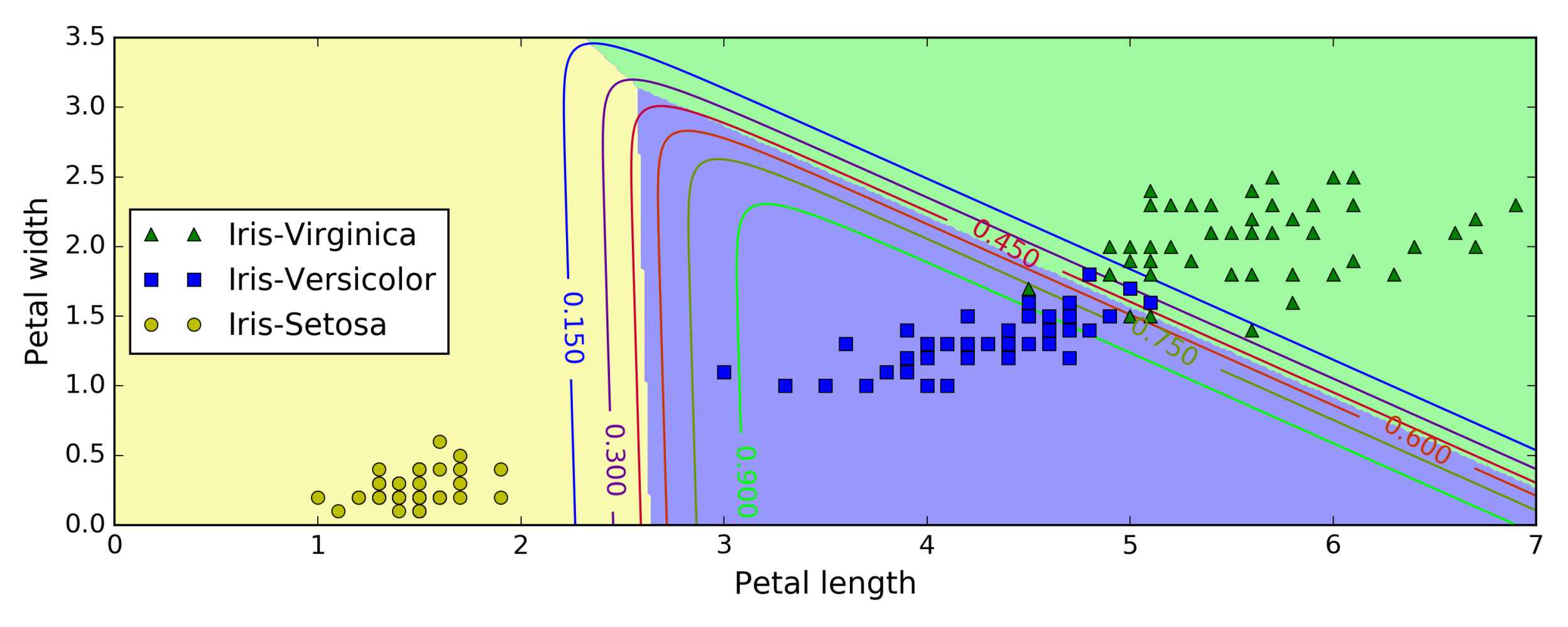## 练习

1. 如果你有一个数百万特征的训练集，你应该选择哪种线性回归训练算法？
2. 假设你训练集中特征的数值尺度（scale）有着非常大的差异，哪种算法会受到影响？有多大的影响？对于这些影响你可以做什么？
3. 训练 Logistic 回归模型时，梯度下降是否会陷入局部最低点？
4. 在有足够的训练时间下，是否所有的梯度下降都会得到相同的模型参数？
5. 假设你使用批量梯度下降法，画出每一代的验证误差。当你发现验证误差一直增大，接下来会发生什么？你怎么解决这个问题？
6. 当验证误差升高时，立即停止小批量梯度下降是否是一个好主意？
7. 哪个梯度下降算法（在我们讨论的那些算法中）可以最快到达解的附近？哪个的确实会收敛？怎么使其他算法也收敛？
8. 假设你使用多项式回归，画出学习曲线，在图上发现学习误差和验证误差之间有着很大的间隙。这表示发生了什么？有哪三种方法可以解决这个问题？
9. 假设你使用岭回归，并发现训练误差和验证误差都很高，并且几乎相等。你的模型表现是高偏差还是高方差？这时你应该增大正则化参数，还是降低它？
10. 你为什么要这样做：
• 使用岭回归代替线性回归？
• Lasso 回归代替岭回归？
• 弹性网络代替 Lasso 回归？
1. 假设你想判断一副图片是室内还是室外，白天还是晚上。你应该选择二个逻辑回归分类器，还是一个 Softmax 分类器？
2. 在 Softmax 回归上应用批量梯度下降的早期停止法（不使用 Scikit-Learn）。

（第一部分 机器学习基础）

（第二部分 神经网络和深度学习）

seancheney
+ 关注